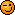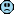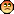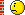Professional Pilot Training (includes ground studies) A forum for those on the steep path to that coveted professional licence. Whether studying for the written exams, training for the flight tests or building experience here's where you can hang out.

# ATPL theory questions

Join Date: Jan 2009
Location: The 3 Valleys
Posts: 187
" I would of went..."

Is it only when they can't tie their shoelaces that they are officially morons ?Join Date: Dec 2006
Location: Hamburg
Age: 43
Posts: 428
thibautg78,

I can't say I have always been successful in my flight school's quality checks, but still I'd like to take an educated guess.
1. LNAV and VNAV can be used simultaneously, and in fact they are during climb and descent. How else would you follow an SID or a STAR? However, VNAV is not usually used during cruise flight. Instead the APFDS is used in height lock, but this does not mean you cannot operate LNAV and VNAV together. So, the correct answer is "b".
2. I'm not certain either. In an A320 an RA failure gives a single chime (i. e. an aural warning) and a master caution light (not a red lamp, as in the question, but still a lamp). Hence, I would tend to "c".
3. The correct answer is "a" (independent mode). In single mode only one FMS is operational. I've never heard of dual or seperate mode.Join Date: Jul 2008
Location: Paris
Age: 32
Posts: 34
Thanks guys, i'll keep you aware of the right answers once i have submittted my on-line assessment.Join Date: May 2001
Posts: 10,817
I think in the majority of old types the first thing you will know about a rad alt failure is the EGPWS going to the wind. Then when you manage to find out where they have fitted the sodding thing on this airframe it will have a red flag on it.

But a word of caution the ATPL's use the 737 as it's generic Jet.

Be a bit careful using Airbus as a reference to answer questions.

On my type the answers would be

D A AJoin Date: Sep 2005
Location: Denmark
Posts: 58
On my type...

hi,

On the A320 the answers would go like this:

1) B - We can and do use LNAV/VNAV together.

2) B - it's an amber light on the airbus

3) A

hope it helps and good luck,

Last edited by Coldbear; 3rd Jan 2010 at 10:34.Join Date: Jun 2002
Location: Wor Yerm
Age: 65
Posts: 0
1. In the UK, HDG is the normal selection. (But I think they are looking for B). FWIW, the use of VNAV on its own is generally not recommended. And on an Embraer E jet, I wouldn't use VNAV at all because it's pants.
2. What a crap question, it is too type and version specific.
3. Again, a type specific question, but A would probably be the best answer.

If I was asked these questions in an exam, I would refuse too answer them as they are too type and version specific.Join Date: Oct 2009
Location: ISRAEL
Posts: 31
Need help with a navigation problem...

HI guys,

Ok, here is the question. I understand how to get the NDB true direction, but for the love of god, i cant understand how they came up with 273 Radial as the one the aircraft has intercepted.. Anyone?

An aircraft maintaining heading 140°(M) at 057°E (VAR 12°E) takes a relative bearing reading of 295° from an NDB station co-located with a VOR at 32°N 088°E (VAR 8°E). Find the true direction of the straight line that should be plotted from the NDB to the aircraft on a Mercator chart. Which radial has the aircraft intercepted at that moment? (Use mean latitude 27°N).Join Date: Oct 2009
Location: ISRAEL
Posts: 31Wow.. 100 + views and no replies...

No worries, I figured it out..

You take the True bearing, add the conversion angle and then convert it to magnetic.

274T + 7 - 8E = 273 Radial.

Now I am stuck with Lambert charts...Join Date: Feb 2002
Location: Sunny Solihull
Age: 64
Posts: 214
Good question, the key bit is understanding for NDBs variation is measured at the aircraft and VORs measured at the VOR. Also straight line on Merc = Rhumb Line. Remember radio bearings follow great circles.

NDB - Hdg(T) = 152 + 295 = 447 -360 = 087(T) from A/C to NDB
As there is more than 2degrees ch long need to apply convergency.

CGY = CH Long (31) x sine 27 (.4540) = 14
Conversation Angle = 14/2 = 7
RL Track from A/C to NDB/VOR = 094 + 180 = 274 to plot on Merc from NDB.

GC Track at NDB/VOR = 087 + CGY (14) = 101 + 180 = 281(T)
281 - 8E at VOR = 273(M) = radial or QDR

Might help to draw a picture and there are a number of ways to do this question.

Ah happy days!Join Date: Oct 2009
Location: ISRAEL
Posts: 31Thank you very much richard. I really appreciate itHere is another problem I got stuck with.. Any idea?

Here is my question:

Following a Rhumb line track starting from E in Lat N050.

E to F 000T, 300NM
F to G 090T, 300NM
G to H 180T, 300NM

What is the Rhumb line bearing and distance of H to E?

The RLB just at a glance seems to be 090... but the distance??

I tried and tried, and then skipped, but would really appreciate it if someone can help.

Thanks...Join Date: Aug 2008
Location: London
Posts: 118
466.7 NM

that's the best I could come up with.

By the way, you will need to use the Departure formula as you are on a latitude of 50 degrees. Hence 60 NM is not 60 minutes.

Departure = change in longitude x Cos Latitude

Change in Longitude in minutes.Join Date: Oct 2009
Location: ISRAEL
Posts: 31
Hi G.

Thanks for trying, but the answer is 336Nm.

300 / cos55 = 523nm

523 x cos50 = 336Nm

Thanks againJoin Date: Feb 2002
Location: Sunny Solihull
Age: 64
Posts: 214

Assume E is at N50 (as given) on prime meridian.
E to F 300 so F is N55 E/W000
F to G 300/cos55/60 = CH long of 8:43 so G N55 E008 43
South to H will bring you to N50 E008 43

I think RL bearing is 270 (but I am a little tired).
8:43 = 523 min * cos 50 = 336 nm.

You must get into drawing diagrams.
Very common type of question and is all about departure and ch long varying with cosine of latitude due to spherical earth.

Members of the flat earth society think you end you back at the start!Join Date: Oct 2009
Location: ISRAEL
Posts: 31Hey Richard,

Ok., here is a new oneThis one seems a little bit more complex.

Two aircraft are converging to the same reporting point at FL 330. The wind is 350 / 60kts. At 0700 the distance between the aircraft is 130NM, and the controller requests aircraft A to reduce the Mach number so there will be 3 minutes separation over the reporting point.

Aircraft A: .76M, TAT = -21c, Track 330(T)
Aircraft B: .79, TAT = -19c, Track 060(T)

1)What is the ETA of aircraft A at the reporting point?
2) What Mach number should aircraft A maintain if the SID temp is -45c

Looking forward to hearing from you.Join Date: Jan 2011
Location: asia
Posts: 103
ATPL theory questions

I need help regarding solving of these questions,i am not able to get the concept to solve these meteorology problem,i would really appreciate if some one could help me out in these concepts

Q1. MSA given as 12000ft, flying over mountains in temperatures +9*c ,QNH set as 1023.what will the true altitude when 12000 ft is reached?
a. 11,940
b. 11,148
c. 12,210
D. 12,864
ANS -D{..........how plz explain.....}

Q2.Flying at FL135above the sea, the Radio altimeter indicates a true altitude of 13500ft. the local QNH is 1019hpa.hence the crossed air mass is on average,

a. At ISAstandard atmospher
b. Colder than ISA
C. Warmer than ISA
D. There is insufficient information
ANS-B{...why plz explain...}

Q3. You are flying at FL160 with an OAT OF -27*C.QNH is 1003 hpa. what is your true altitude?
a.15540 ft
b.15090 ft
c.16330 ft
d.15730 ft
ANS-B{.....how plz explain.....}

Q4. What is approximate vertical interval which is equal to a pressure change of 1hpa at an altitude of 5500m?
a. 8m{27ft}
b. 32m{105ft}
c. 64m{210ft}
d. 15m{50ft}
ANS-D{....how plz explain...}.

thanksJoin Date: Mar 2010
Location: Not from round these parts
Posts: 20
Q1. True altitude differs from indicated by 4% for every 10 degrees difference in temperature from ISA. True altitude is higher than indicated in warm air, lower in cold air. At 12,000 ft ISA temperature is -9 degrees, if the actual temperature is +9 then the temperature is ISA+18, so 1.8 x 4 = 7.2 percent difference, the actual altitude is 107.2% of the indicated.

Q2. If the QNH was 1013mb, you'd be at 13,500 feet when at FL135. Since if you increased the subscale setting to 1019 the indication would increase, then FL135 is actually a bit lower than 13,500 ft. (You're flying into higher pressure air than ISA, so you're higher than you would be if it were ISA). Since the radio altimeter says you are really at 13,500 feet, you must be in warmer than ISA air.

Q3. FL160 is 15,730 feet above sea level if the QNH is 1003. (FL measures from 1013 and since the pressure at sea level is 1003 the datum you are measuring from is below sea level). OAT is -27 wheras ISA temperature at your level would be -17, so it's ISA-10, so take off 4% to get 15100ft - 15090 is close enough.

Q4. 50ft and you just have to memorise these, although you could make a decent guess if you knew it was 27ft at sea level and about 100ft at airliner cruising levels, then 5500m=16000 odd feet will be somewhere in the middle.Join Date: Jan 2005
Location: UK
Posts: 2
Altimeter Calculations

Use the following formulas for Altimeter calculations

TA = IA + (4 x IA/1000 x ISA Dev) + (27 x (QNH  Subscale))

where TA = true altitude, IA = indicated altitude, Subscale = altimeter subscale setting, ISA Dev = ISA temperature deviation (I assume you can calculate this). This will work for the vast majority of all altimetry questions, except PPL questions use 30ft (not 27).

This will give you the exact answers to questions 1, and 3. I think you mean the indicated altitude at an MSA (true) of 12000ft in Q1.

Question 2 is slightly flawed in that radio altimeters operate only up to 2500ft, and actually read height above the surface directly below rather than altitude. If you put this aside you can determine that the ISA dev is -ve, ie colder using the equation above.

The following formula

Height change per hPa(ft)= 96 x T/P

where T= temperature in Kelvin (C + 273), P = Pressure in hPa

In Q4, T= 252K, ISA in C is -21, as 5500m is approximately 18000ft, P = 500hPa

That gives
Height change per hPa = 96x252/500 = 48.4ftJoin Date: Jan 2011
Location: asia
Posts: 103
meteorology question

Q. you intend to overfly a mountain ridge at an altitude of 15000ft AMsL.The average air temp i s 15degree lover than IsA.the sealevel pressure 1003Hpa.which altimeter indication is needed?

Ans--16170ft...............plz explain hw to solve this problem
thanksJoin Date: Mar 2008
Location: N/A
Posts: 2
Q. You intend to overfly a mountain ridge at an altitude of 15000ft AMSL.The average air temperature (=ISA Deviation) is 15 degree lower than ISA. The Sealevel Pressure (=QNH)1003Hpa. Which altimeter indication is needed?

15 x 15 x 4 = 900
1013 - 1003 = 10 x 27 = 270

900 + 270 = 1170

15000 + 1170 = 16170

Read Angleofattacks post.. and use the formulae
TA = IA + (4 x IA/1000 x ISA Dev) + (27 x (QNH  Subscale))Join Date: Oct 2011
Posts: 3
Drift and True altitude

Hello guys,

I am currently studying for my cdn atpl and I came across a MET question I cannot seem to grasp. The concept of port/starboard drift increasing/decreasing true altitude respectively. Now the in the spirit of trying to understand the subject rather than simply memorizing the port drift=increasing true alt and starboard drift=decreasing true altitude, I am trying to attempt to understand this concept. Now where I get mixed up is how do you know whats happening because you could get either port or starboard drift depending on where you sit around the low or the high right? Isn't it a matter of perspective? eg port drift: I could be to the east of a low or west of a high if i was on a west east trip right???Show Printable VersionEmail this Page